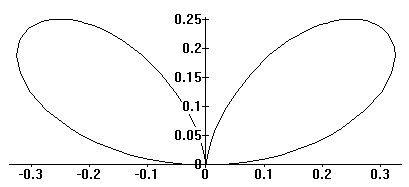MAT37x
Franklin College
Spring2004
Erich Prisner,

# Functions R ---> R3

We have one independent variable, usually denoted, t for time, and f(t) is a vector in three-dimensional space. Thus f(t)=(f1(t),f2(t),f3(t))---we call it the coordinates of a point at time t. We call the equations  x=f1(t), y=f2(t), z=f3(t) parametric, where  t is the parameter.

## Examples:

NamePictureEquations
Astroidx=a cos3(t)y=a sin3(t) x2/3+y2/3=a2/3
Cardioidr = 2a(1+sin(Q)) (x2+y2-2ay)2 = 4a2(x2+y2)
Double Foliumr = 4a sin(Q) cos(Q2) (x2+y2)2 = 4ax2y

## Velocity, Acceleration

The velocity of the point is the derivative of f, i,e, the function f'(t) = (f1'(t),f2'(t),f3'(t)). The speed is the absolute value of the velocity. The acceleration is the second derivative f''(t) = (f1''(t),f2''(t),f3''(t)) of  f.

Let T=f'(t)/|f'(t)| denote the unit tangent vector for time  t